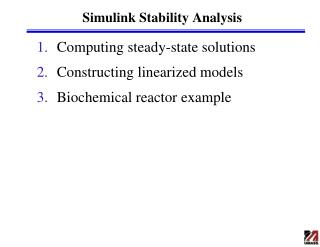DownloadDownload Presentation# Simulink Stability Analysis

Download Presentation## Simulink Stability Analysis

- - - - - - - - - - - - - - - - - - - - - - - - - - - E N D - - - - - - - - - - - - - - - - - - - - - - - - - - -
##### Presentation Transcript

1. Simulink Stability Analysis • Computing steady-state solutions • Constructing linearized models • Biochemical reactor example

2. Computing Steady-State Solutions • Matlab function trim – finds steady state solutions for a Simulink system >> [x,u,y,dx]=trim(sys,x0,u0) • Attempts to find values for x, u and y that set the state derivatives, dx, of the S-function sys to zero using a constrained optimization technique. • Sets the initial starting guesses for x and u to x0 and u0, respectively.

3. Computing Linearized Models • Matlab function linearize – obtains a linear model from a Simulink model >> linsys = linearize(sys,sys_io) • Takes a Simulink model, sys, and an I/O object, sys_io, as inputs and returns a linear state-space model, linsys. The linearization I/O object is created with the function linio. >> sys_io=linio(blockname,portnum,type) • Creates a linearization I/O object for the signal that originates from the outport with port number, portnum, of the type given by type of the block, blockname, in a Simulink model. Available linearization I/O types include: 'in', linearization input point 'out', linearization output point

4. Biochemical Reactor Example • Continuous bioreactor model • Parameter values • KS = 1.2 g/L, mmax = 0.48 h-1, YX/S = 0.4 g/g • D = 0.15 h-1, Si = 20 g/L • Steady-state solutions • Eigenvalues

6. S-Function function [sys,x0] = bioreactor_basic(t,x,u,flag) % % Mike Henson, September 23, 2010 % %% Parameters Ks=1.2; Yxs=0.4; mumax=0.48; Si=20.0; D=u; switch flag, %% Derivatives case 1, X=x(1); S=x(2); mu=mumax*S/(Ks+S); dxdt = [-D*X + mu*X; D*(Si-S)-mu*X/Yxs]; sys = dxdt;

7. S-Function cont. %% Outputs case 3, X=x(1); Y=x(2); y = X; sys = y; %% Initialization case 0, NumContStates = 2; NumOutputs = 1; NumInputs = 1; sys = [NumContStates,0,NumOutputs,NumInputs,0,0]; x0 = [7.78 0.545];

8. S-Function cont. %% Unhandled flags case { 2, 4, 9 }, sys = []; %% Unexpected flags error(['Unhandled flag = ',num2str(flag)]); end

9. In-Class Exercise • Use the Matlab function trim to find the two steady-state solutions • Use the Matlab function linearize to find a linear state-space model at the non-trivial steady state • Use the Matlab function eig to check the stability of the non-trivial steady state • Use the Matlab function ss2tf to construct the transfer function from the linear state-space model

10. Steady-State Solutions >> sys = 'bioreactor_stability'; >> load_system(sys); >> open_system(sys); >> [x1,u1,y1,dx1]=trim(sys,[1; 1],[]); >> x1 x1 = 7.7818 0.5455 >> [x2,u2,y2,dx2]=trim(sys,[0; 0],[]); >> x2 x2 = 0.0000 20.0000

11. Linear Model Analysis >> sys_io(1)=linio('bioreactor_stability/Dilution',1,'in'); >> sys_io(2)=linio('bioreactor_stability/Bioreactor',1,'out'); >> linsys = linearize(sys,sys_io) a = Bioreactor(1 Bioreactor(2 Bioreactor(1 -8.596e-005 1.472 Bioreactor(2 -0.3748 -3.829 b = Dilution (pt Bioreactor(1 -7.78 Bioreactor(2 19.45 c = Bioreactor(1 Bioreactor(2 bioreactor_s 1 0 d = Dilution (pt bioreactor_s 0 >> lambda=eig(linsys.a) lambda = -0.1500 -3.6793

12. Transfer Function >> [num,den] = ss2tf(a,b,c,d,iu) • Calculates the numerator num and denominator den polynomials of the transfer function for the iu-th input given the matrices a, b, d and d of the state-space system >> g = tf(num,den) • Forms the transfer function g from the numerator and denominator polynomials >> [num,den] = ss2tf(linsys.a,linsys.b,linsys.c,linsys.d,1) num = 0 -7.7800 -1.1596 den = 1.0000 3.8293 0.5519 >> g = tf(num,den) Transfer function: -7.78 s - 1.16 ---------------------- s^2 + 3.829 s + 0.5519